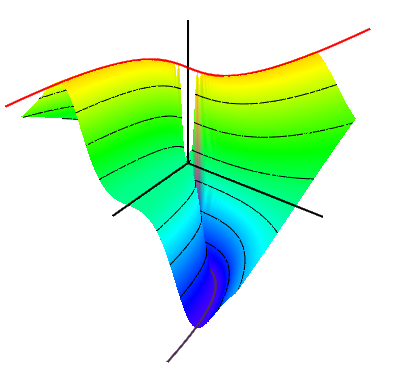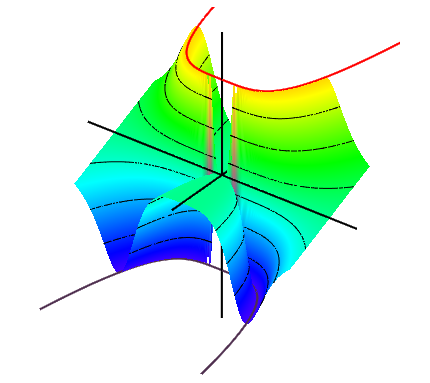# One of my favorite counterexamples

Differentiability in two variables is one of those especially challenging concepts in Multivariable Calculus.   Its definition is a challenge even for seasoned mathematicians, much less your typical undergraduate student (this will in fact be the subject of an upcoming post/question for the math folks out there).   Moreover, multivariable differentiability seems to subscribe to Finagle’s Law: Anything that can go wrong, will.

What is interesting, however, is that many examples of the things that go wrong can be found by playing around with just one function, specifically, the function$displaystyle f(x,y) = left{ begin{array}{ll} displaystyle frac{2xy}{x^2+y^2}, & (x,y) neq (0,0) & 0, & (x,y)=(0,0) end{array} right.$.

Here’s a graph of$f$:So what dastardly deeds does this function$f$ commit against differentiability?   Read on to find out!

Discussion 1: Limits. You might recognize this function already, as it often turns up in calculus books during the discussion about limits, since it has the property that while the limit$displaystyle lim_{(x,y) to (0,0)} f(x,y)$ does not exist, the function$f$ does have a limit at the origin when restricted to any straight line.   More precisely, note that$displaystyle lim_{t to 0} f(at, bt) = frac{2ab}{a^2 + b^2}$,

which implies that when$f$ is restricted to the line passing through the point$(a,b)$ and the origin, it has a constant value of$2ab/(a^2 + b^2)$.

In particular, when restricted to either the$x$ or$y$ axes (so that either$a=0$ or$b=0$), then the limiting value is 0.   On the other hand, when restricted to the line$y=x$ (so that$a=b=0$), then the limiting value is 1 (this is the red line on the graph above).   In particular,$f$ has no limit at the origin.

Discussion 2: Partial derivatives don’t imply differentiability. The function$f$ also typically turns up during the discussion about partial derivatives.   In particular, the existence of both partial derivatives at a point is insufficient to prove that a function is even continuous at that point, much less differentiable.   To see this, imply note that$f(x,0) equiv f(0,y) equiv 0$,

which implies that$displaystyle frac{partial f}{partial x}(0,0) = frac{partial f}{partial y}(0,0) = 0$.

Hence, both partial derivatives exist at the origin, but the previous discussion shows that$f$ is not continuous there.

(In fact,$f$ shows something stronger — it has a pair of well-defined partial derivatives at every point in the plane, and yet still is discontinuous at the origin.)

Discussion 3. Directional derivatives don’t imply differentiability. Sharp students will often note that the existence of just two “directional derivatives” should be insufficient to conclude differentiability in the same way that just two “directional limits” agreeing should be insufficient to conclude a 2-variable limit exists.   This is definitely the case with the function$f$ — it does not have a directional derivative in any direction not parallel to one of the axes.

However, consider instead the function$f_1(x,y) = f(x^2,y)$, pictured below.Note that when restricted to the line through the origin and the point$(a,b)$, we find$displaystyle f_1(ta,tb) = frac{2a^2b , t}{a^2 , t^4 + b^2}$.*

In particular, if$vec{u} = (a,b)$ is a unit-length vector, then the directional derivative of$f_2$ in the direction$vec{u}$ at the origin is$displaystyle nabla_{vec{u}} f_1(0,0) = lim_{t to 0} frac{f_1(ta,tb)-0}{t} = frac{2 a^2}{b}$

if$b neq 0$ (and equals 0 otherwise).   Hence,$f_1$ has directional derivatives at the origin in every direction.   Nevertheless, it is still discontinuous, since$f_1$ restricted to the curve$y = x^2$ has constant value$1$… except at the origin.

* As a bonus,$f_1$ has a second cool property. Observe that$displaystyle lim_{t to 0} f_1(ta,tb) = lim_{t to 0} frac{2a^2b , t}{a^2 , t^4 + b^2} = 0$.

In particular,$f_1$ has a limiting value of$0$ at the origin when restricted to every line passing through the origin, and yet still fails to have a limit at the origin.

Discussion 4. Directional derivatives and the Chain Rule still don’t imply differentiability. This might take a moment to explain.   If a function$g$ is differentiable at a point$(x_0,y_0)$, then its directional derivative can be determined by the Chain Rule as$nabla g_{vec{u}}(x_0,y_0) = nabla g(x_0,y_0) cdot vec{u}$.

The directional derivatives of the function$f_1$ from the previous discussion clearly do not satisfy this property, as$nabla f_1(0,0) = vec{0}$, but the directional derivatives of$f_1$ are nonzero for any direction not parallel to either axis.

A reasonable hypothesis might be that if a function had directional derivatives in all directions at a point and they satisfied the Chain Rule, then the function would be differentiable.     (This is certainly true in one variable!)

Unfortunately, this is not to be… consider the function$f_2(x,y) = f(x^3,y)$, pictured below:As in the previous case, observe that when$f_2$ is restricted to the line through the origin and the point$(a,b)$, we find$displaystyle f_2(ta,tb) = frac{2a^3b , t^2}{a^6 , t^4 + b^2}$.

In particular, if$vec{u} = (a,b)$ is a unit-length vector, then the directional derivative of$f_2$ in the direction$vec{u}$ at the origin is$displaystyle nabla_{vec{u}} f_2(0,0) = lim_{t to 0} frac{h(ta,tb)-0}{t} = 0$.

Hence,$f_2$ has directional derivatives of 0 at the origin in every direction, so it satisfies (rather trivially) the Chain Rule at the origin.   Yet$f_2$ is still discontinuous, since when restricted to the curve$y = x^3$ it has constant value 1… except at the origin.

Discussion 5. In fact, it’s much, much worse than Discussion 4! It’s an easy argument to show that the hypothesis in the previous discussion is equivalent to the following statement: “If$g$ restricted to any line vanishes at the origin of order 2, then$g$ is differentiable at the origin, (necessarily with$nabla g(0,0) = vec{0}$). ” Can the hypothesis be strengthened enough to ensure this conclusion?

For example, suppose that$g$ vanishes at the origin of order (at least) 3 when restricted to any line, that is, that$g(ta,tb) = t^3 a(t)$, where the function$a(t)$ is bounded near the origin.   Does this ensure$g$ is differentiable?

No.   Just consider the function$f_3(x,y) = f(x^4,y)$, pictured below:This would be the desired counterexample.   To prove this, let’s in fact consider something more general.

Define$f_n(x,y) = f(x^{n+1},y)$, where$n ge 1$ is an integer.   A simple calculation shows that$displaystyle f_n(ta,tb) = frac{2a^{n+1}b , t^n}{a^{2(n+1)} t^{2n} + b^2}$,

so that$f_n$ vanishes at the origin of order$n$ on any line through the origin.   Nevertheless,$f_n$ is discontinuous at the origin, since$f_n$ restricted to the curve$y=x^{n+1}$ has constant value 1 … except at the origin.

But it’s even worse than this!   It’s possible for a function$g$ to vanish at the origin of infinite order when restricted to any line segment and still fail to muster even continuity!   Consider the function$f_infty$ defined as$f_infty(x,y) = f big( e^{-1/x^2}, y big)$ for nonzero$x$, and$f_infty(0,y) = 0$; this is shown below.Note that$f_infty$ clearly vanishes to infinite order along the x-axis.   Moreover, when restricted to the line through the origin and the point$(a,b)$ off the x-axis,$f_infty$ becomes$displaystyle f_infty(ta,tb) = left{ begin{array}{ll} displaystyle frac{2b , t , e^{-1/a^2t^2}}{e^{-2/a^2t^2}+b^2,t^2}, & (x,y) neq 0 & 0, & (x,y)=(0,0) end{array} right.$.

Since L’Hospital’s Rule implies that$displaystyle lim_{t to 0} frac{ e^{-1/a^2 x^2}}{t^p} = 0$ for any integer$p ge 0$, it follows that this function vanishes at the origin to any order whatsoever.

Nevertheless, when restricted to the curve$y=e^{-1/x^2}$, the function has constant value 1, which once again implies that$f_infty$ cannot be continuous at the origin.

Discussion 6. The differentiability of functions of 2 real variables is intimately connected with the differentiability of functions of 1 complex variable, in that the former is a necessary, but not sufficient, condition for the latter.   A typical example of a function that is differentiable in the real sense, but not in the complex one, is the conjugation function$c(z) = overline{z}$.     In proving that this is not complex differentiable, we would compute$displaystyle frac{dc}{dz}(z_0) = lim_{z to 0} frac{ overline{z_0 + z} - overline{z_0} }{ z } = lim_{z to 0} frac{ , overline{z} , }{z}$.

But setting$z = x + i, y$, a quick calculation shows that$displaystyle frac{ , overline{z} , }{z} = frac{x - i , y}{x + i, y} = f(x+y,x-y) + i , f(x,y)$,

where$f$ is our favorite counterexample!   In particular, this calculation shows that the complex function$w(z) = overline{z}/z$ has no limit as$(x,y) to 0$, which means that the conjugation function is not complex differentiable at any point in the plane, despite being (infinitely!) differentiable in terms of the real variables$(x,y)$.

This entry was posted in mathify. Bookmark the permalink.